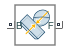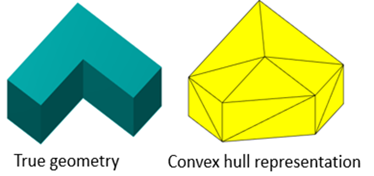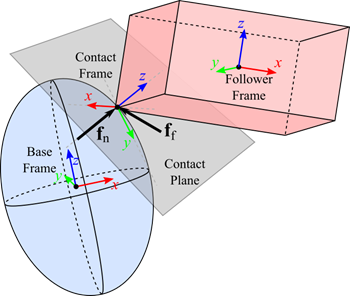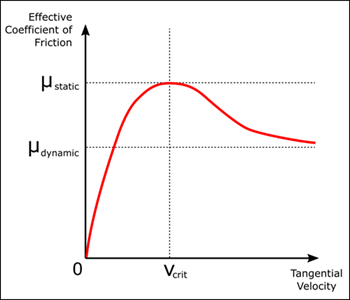# Spatial Contact Force

Model spatial contact between two geometries

Since R2019b

•Libraries:
Simscape / Multibody / Forces and Torques

## Description

The Spatial Contact Force block models the contact between a pair of geometries in 3-D space. You can use the built-in penalty method or provide custom normal and friction force laws to model a contact.

### Supported Geometries

The Spatial Contact Force block can model contacts between a variety of geometry pairs. You can use the geometries exported from the solid blocks in the Body Elements sublibrary or the geometries of the point and surface blocks in the Curves and Surfaces sublibrary.

All the exported geometries are convex hull representations of the corresponding solids even though some of the solids may have concave shapes. The figure shows the true geometry and convex hull representation of an L-shape solid.Note that when computing inertial properties, the solid blocks use the true geometry.

The Spatial Contact Force block does not model contacts between certain geometry pairs. This table lists the supported pairs.

 Convex Hull of Solid Disk Grid Surface Infinite Plane Point Point Cloud Convex Hull of Solid Yes Yes No Yes Yes Yes Disk Yes No No Yes No No Grid Surface No No No No Yes Yes Infinite Plane Yes Yes No No Yes Yes Point Yes No Yes Yes No No Point Cloud Yes No Yes Yes No No

### Contact Forces

The image shows how the Spatial Contact Force block models a spatial contact problem between two 3-D geometries. In this case, the contact is between a blue base geometry and a red follower geometry and there is one contact point.During contact, each geometry has a contact frame. The contact frames are always coincident and located at the contact point. The xy-planes of the contact frames define the contact plane. The z-direction of the contact frames is an outward normal vector for the base geometry, but an inward normal vector for the follower geometry. During continuous contact, the contact frames move around the geometry as the contact point moves.

The block applies contact forces to the geometries at the origin of the contact frames in accordance with Newton's Third Law:

1. The normal force, ${f}_{n}$, which is aligned with the z-axis of the contact frame. This force pushes the geometries apart in order to reduce penetration.

2. The frictional force, ${f}_{f}$, which lies in the contact plane. This force opposes the relative tangential velocities between the geometries.

To specify a normal contact force, in the Normal Force section, set the Method parameter to `Smooth Spring-Damper` or `Provided by Input`. If you select ```Smooth Spring-Damper```, the normal force is:

${f}_{n}=s\left(d,w\right)\cdot \left(k\cdot d+b\cdot {d}^{\text{'}}\right)$,

where:

• ${f}_{n}$ is the normal force applied in equal-and-opposite fashion to each contacting geometry.

• $d$ is the penetration depth between two contacting geometries.

• $w$ is the transition region width specified in the block.

• ${d}^{\text{'}}$ is the first time derivative of the penetration depth.

• $k$ is the normal-force stiffness specified in the block.

• $b$ is the normal-force damping specified in the block.

• $s\left(d,w\right)$ is the smoothing function.

The force law is smoothed near the onset of penetration. When d < w, the smoothing function increases continuously and monotonically over the interval [0, w]. The function is 0 when d = 0, the function is 1 when d = w, and the function has zero derivative with respect to d at the endpoints of the interval.

To better detect contacts when the value of the parameter is small, the Spatial Contact Force block supports optional zero-crossing detection. The zero-crossing events only occur when the separation distance changes from positive or zero to negative and vice versa.

Note

The zero-crossing detection of the Spatial Contact Force block is different than the zero-crossing detection of other Simulink® blocks, such as From File and Integrator, because the force equation of the Spatial Contact Force is continuous. For more information about zero-crossing detection in Simulink blocks, see Zero-Crossing Detection.

The Spatial Contact Force block clips the computed force to be always nonnegative. If the force law gives a negative force, the block applies zero force instead. This happens briefly as the geometries are separating and penetration is about to end. At that point, d is approaching zero and d' is negative. This modification ensures that the contact normal force is always repulsive and never attractive.

To specify a frictional force, in the Frictional Force section, set the Method parameter to `Smooth Stick-Slip`, `Provided by Input`, or `None`. If you select `Smooth Stick-Slip`, the frictional force is always directly opposed to the direction of the relative velocity at the contact point and is related to the normal force through a coefficient of friction that varies depending on the magnitude of the relative velocity:

$|{f}_{f}|=\mu \cdot |{f}_{n}|$,

where:

• ${f}_{f}$ is the frictional force.

• ${f}_{n}$ is the normal force.

• $\mu$ is the effective coefficient of friction.

The effective coefficient of friction is a function of the values of the Coefficient of Static Friction, Coefficient of Dynamic Friction, and Critical Velocity parameters, and the magnitude of the relative tangential velocity. At high relative velocities, the value of the effective coefficient of friction is close to that of the coefficient of dynamic friction. At the critical velocity, the effective coefficient of friction achieves a maximum value that is equal to the coefficient of static friction. The graph shows the basic relationship in the typical case where ${\mu }_{static}$ > ${\mu }_{dynamic}$. In this case, the model is able to approximate stiction with a higher effective coefficient of friction near small tangential velocities.When modeling contacts that involve a point cloud, the Spatial Contact Force block calculates the contact quantities for each point and the output signals have the same order as the points specified in the Point Could block. For the points that are not in contact with the other geometry, the measured values are zero. When using input forces, the size and order of the input signals must match the size and order of the points specified in the Point Could block.

## Ports

### Geometry

expand all

Geometry port associated with the base geometry.

Geometry port associated with the follower geometry.

### Input

expand all

Physical signal input port that accepts the normal contact force magnitude between the two geometries. The block clips negative values to zero.

When modeling contacts that involve a point cloud, the input signal must be a 1-by-N array, where N equals the number of points. Each column of the array specifies the normal contact force on one of the points. The size and order of the input signals must match the size and order of the points specified in the Point Could block. The force is resolved in the contact frame of the point.

When modeling contacts between other types of geometries, the input signal must be a scalar that specifies the normal contact force.

#### Dependencies

To enable this port, in the Normal Force section, set Method to ```Provided by Input```.

Physical signal input port that accepts the frictional force between the two geometries.

When modeling contacts that involve a point cloud, the input signal must be a 2-by-N matrix, where N equals the number of points. Each column of the matrix specifies the x-component and y-component of the applied frictional force on one of the points. The size and order of the input signals must match the size and order of the points specified in the Point Could block. The force is resolved in the contact frame of the point.

When modeling contacts between other types of geometries, the input signal must be a 2-by-1 array that specifies the x-component and y-component of the applied frictional force resolved in the contact frame.

#### Dependencies

To enable this port, in the Frictional Force section, set Method to ```Provided by Input```.

### Output

expand all

Physical signal output port that outputs the contact status of the two geometries.

When modeling contacts that involve a point cloud, the output is a 1-by-N array, where N equals the number of points. Each column of the array indicates the status of the corresponding point. The point contacts the other geometry if the value is 1. Otherwise, the value is 0.

When modeling contacts between other types of geometries, the output is a scalar that indicates whether the geometries are in contact. The geometries are in contact if the output is 1 or separated if the output is 0.

#### Dependencies

To enable this port, in the Sensing section, select Contact Signal.

Physical signal output port that outputs the penetration depth between the two geometries.

When modeling contacts that involve a point cloud, the output is a 1-by-N array, where N equals the number of points. Each column of the array indicates the status of the corresponding point. If the point is penetrating the other geometry, the value is positive and equals the penetration depth. Otherwise, the value is 0.

When modeling contacts between other types of geometries, the output is a scalar that indicates whether the geometries are penetrating each other. If they are penetrating each other, the output is positive. Otherwise, the output is 0.

#### Dependencies

To enable this port, in the Sensing section, select Penetration Depth.

Physical signal output port that outputs the separation distance between the two geometries.

When modeling contacts that involve a point cloud, the output is a 1-by-N array, where N equals the number of points. Each column of the array indicates the status of the corresponding point. If the point is not penetrating the other geometry, the value is nonnegative and equals the minimum distance between the point and the other geometry. Otherwise, the output is negative and the absolute value equals the penetration depth.

When modeling contacts between other types of geometries, the output is a scalar. If they are not penetrating each other, the output is nonnegative and equals the minimum distance between the two geometries. Otherwise, the output is negative and the absolute value equals the penetration depth.

#### Dependencies

To enable this port, in the Sensing section, select Separation Distance.

Physical signal output port that outputs the magnitude of the normal contact force between the two geometries. The output is always nonnegative.

When modeling contacts that involve a point cloud, the output is a 1-by-N array, where N equals the number of points. Each column of the array equals the magnitude of the normal contact force on the corresponding point.

When modeling contacts between other types of geometries, the output is a scalar that equals the magnitude of the normal contact force.

#### Dependencies

To enable this port, in the Sensing section, select Normal Force Magnitude.

Physical signal output port that outputs the magnitude of the frictional contact force between the two geometries.

When modeling contacts that involve a point cloud, the output is a 1-by-N array, where N equals the number of points. Each column of the array equals the magnitude of the frictional force on the corresponding point.

When modeling contacts between other types of geometries, the output is a scalar that equals the magnitude of the frictional force.

#### Dependencies

To enable this port, in the Sensing section, select Frictional Force Magnitude.

Physical signal output port that provides the z-component of the relative velocity between the contact points of the base and follower geometries.

When modeling contacts that involve a point cloud, the output is a 1-by-N array, where N equals the number of points. Each column of the array equals the z-component of the relative velocity between the corresponding point and the contact point on the other geometry. The relative velocity is resolved in the corresponding contact frame.

When modeling contacts between other types of geometries, the output is a scalar that equals the z-component of the relative velocity between the contact points of the two geometries. The output is resolved in the contact frame.

#### Dependencies

To enable this port, in the Sensing section, select Relative Normal Velocity.

Physical signal output port that outputs the relative tangential velocity between the contact points of the base and follower geometries.

When modeling contacts that involve a point cloud, the output is a 2-by-N matrix, where N equals the number of points. Each column of the array equals the x-component and y-component of the relative velocity between the corresponding point and the contact point on the other geometry. The relative velocity is resolved in the corresponding contact frame.

When modeling contacts between other types of geometries, the output is a 2-by-1 array that equals the x-component and y-component of the relative velocity between the contact points of the two geometries. The output is resolved in the contact frame.

#### Dependencies

To enable this port, in the Sensing section, select Relative Tangential Velocity.

Contact Frame

Physical signal port that outputs a 3-by-3 rotation matrix that maps the vectors in the contact frame to vectors in the reference frame of the base geometry. The output signal is resolved in the reference frame associated with the base geometry.

When one of the geometries is a point cloud, the Spatial Contact Force block cannot output signals from this port.

#### Dependencies

To enable this port, in the Sensing > Contact Frame section, select Base Rotation.

Physical signal port that outputs the location of the contact point with respect to the reference frame of the base geometry.

When modeling contacts that involve a point cloud, the output is a 3-by-N array, where N equals the number of points. Each column of the array indicates the coordinates of a point with respect to the reference frame of the base geometry. If a point is not in contact with the other geometry, the coordinates are `[0 0 0]`.

When modeling contacts between other types of geometries, the output is a 3-by-1 array that contains the coordinates of the contact point with respect to the reference frame of the base geometry.

#### Dependencies

To enable this port, in the Sensing > Contact Frame section, select Base Translation.

Physical signal port that outputs a 3-by-3 rotation matrix that maps vectors in the contact frame to vectors in the reference frame of the follower geometry. The output signal is resolved in the reference frame associated with the follower geometry.

When one of the geometries is a point cloud, the Spatial Contact Force block cannot output signals from this port.

#### Dependencies

To enable this port, in the Sensing > Contact Frame section, select Follower Rotation.

Physical signal port that outputs the location of the contact point with respect to the reference frame of the follower geometry.

When modeling contacts that involve a point cloud, the output is a 3-by-N array, where N equals the number of points. Each column of the array indicates the coordinates of a point with respect to the reference frame of the follower geometry. If a point is not in contact with the other geometry, the coordinates are `[0 0 0]`.

When modeling contacts between other types of geometries, the output is a 3-by-1 array that contains the coordinates of the contact point with respect to the reference frame of the follower geometry.

#### Dependencies

To enable this port, in the Sensing > Contact Frame section, select Follower Translation.

## Parameters

expand all

### Normal Force

Method to specify the normal contact force, specified as either `Smooth Spring-Damper` or ```Provided by Input```.

Select `Smooth Spring-Damper` to use the modified spring-damper method to model the normal contact force, or select `Provided by Input` to input a custom force as the normal contact force.

Resistance of the contact spring to geometric penetration, specified as a scalar. The spring stiffness is constant during the contact. The larger the value of the spring stiffness, the harder the contact between the geometries.

#### Dependencies

To enable this parameter, in the Normal Force section, set Method to ```Smooth Spring-Damper```.

Resistance of the contact damper to motion while the geometries are penetrating, specified as a scalar. The damping coefficient is a constant value that represents the lost energy from colliding geometries. The larger the value of the damping coefficient, the more energy is lost when geometries collide and the faster the contact vibrations are dampened. Use a value of zero to model perfectly elastic collisions, which conserve energy.

#### Dependencies

To enable this parameter, in the Normal Force section, set Method to ```Smooth Spring-Damper```.

Region over which the spring-damper force raises to its full value, specified as a scalar. The smaller the region, the sharper the onset of contact and the smaller the time step required for the solver. Reducing the transition region improves model accuracy while expanding the transition region improves simulation speed.

#### Dependencies

To enable this parameter, in the Normal Force section, set Method to ```Smooth Spring-Damper```.

### Frictional Force

Method to specify the frictional force, specified as ```Smooth Stick-Slip```, `None`, or ```Provided by Input```.

Select `None` to omit friction, ```Smooth Stick-Slip``` to use the modified stick-slip method to compute the frictional force, or `Provided by Input` to input a custom frictional force.

Ratio of the magnitude of the frictional force to the magnitude of the normal force when the tangential velocity is close to zero, specified as a positive scalar.

This value is determined by the material properties of the contacting geometries. The value of this parameter is often less than one, although values greater than one are possible for high-friction materials. In most cases, this value should be higher than the coefficient of dynamic friction.

#### Dependencies

To enable this parameter, in the Frictional Force section, set Method to ```Smooth Stick-Slip```.

Ratio of the magnitude of the frictional force to the magnitude of the normal force when the tangential velocity is large, specified as a nonnegative scalar.

This value is determined by the material properties of the contacting geometries. The value of this parameter is often less than one, although values greater than one are possible for high-friction materials. In most cases, this value should be less than the coefficient of static friction.

#### Dependencies

To enable this parameter, in the Frictional Force section, set Method to ```Smooth Stick-Slip```.

Velocity that determines the blending between the static and dynamic coefficients of friction, specified as a scalar.

When the critical velocity is equal to the magnitude of the tangential velocity, the effective coefficient of friction is equal to the value of the Coefficient of Static Friction parameter. As the magnitude of the tangential velocity increases beyond the specified critical velocity, the effective coefficient of friction asymptotically approaches the value of the Coefficient of Dynamic Friction parameter.

#### Dependencies

To enable this parameter, in the Frictional Force section, set Method to ```Smooth Stick-Slip```.

### Zero-Crossings

Select to detect the start and end of each contact as zero-crossing events. The zero-crossing events occur when the separation distance changes from positive or zero to negative and vice versa.

## Version History

Introduced in R2019b

expand all